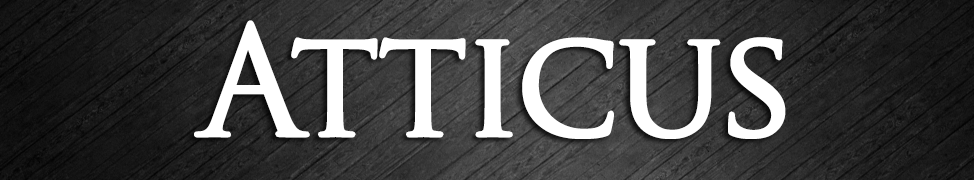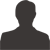90 weeks ago\r
by Mary Ray Birdwell\r
\r
\r
\r
\r
\r
\r
\r
\r
\r
\r
\r
\r
\r
\r
\r
\r
\r
\r
\r
\r
\r
\r
\r
\r
\r
Fading Image Mary Ray Birdwell\r
\r
\r
\r
\r
\r\r
\r